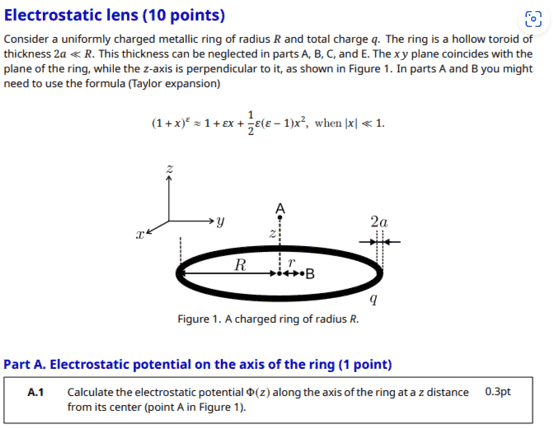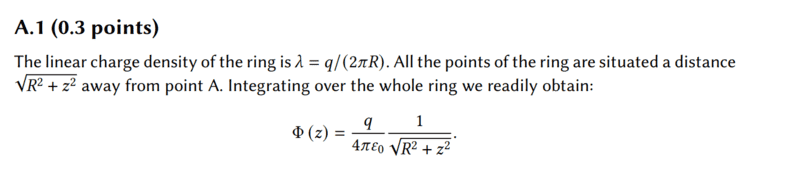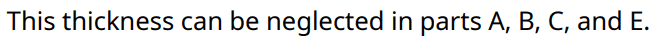# Finding the electric potential above a charged metal toroid (electrostatic lens)

Callumnc1
Homework Statement:
Relevant Equations:
For A.1 of this problem,The solution isHowever, I have a doubt about the linear charge density ##\lambda##.

I don't understand how ##\lambda = \frac {q}{2\pi R} ## since this is not a thin ring, but has a non-negligible width of ##2a##

I think that the toroid has a larger area than thin circle with a circumference ##2\pi R## so linear charge density should be less than that expression.

EDIT: How would we calculate the electric potential if the thickness was not neglected?

Many thanks !

Problem from:
https://www.ipho2021.lt/uplfiles/Th2.pdf
https://www.ipho2021.lt/uplfiles/Th2-Solution.pdf

#### Attachments

Last edited:

Homework Helper•Callumnc1
Callumnc1

However, how would we calculate the electric potential if the thickness was not neglected?

Many thanks!

Homework Helper
Gold Member
2022 Award

However, how would we calculate the electric potential if the thickness was not neglected?

Many thanks!
Since it is metallic, your first challenge would be to figure out the charge distribution. Good luck with that.

•Callumnc1, nasu and BvU
Callumnc1
Since it is metallic, your first challenge would be to figure out the charge distribution. Good luck with that.